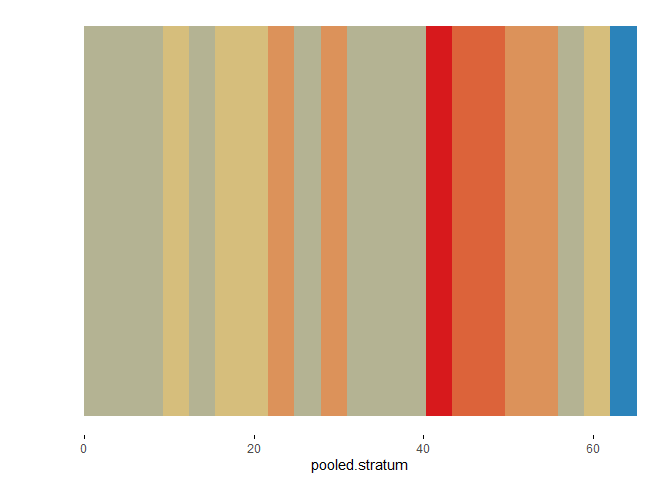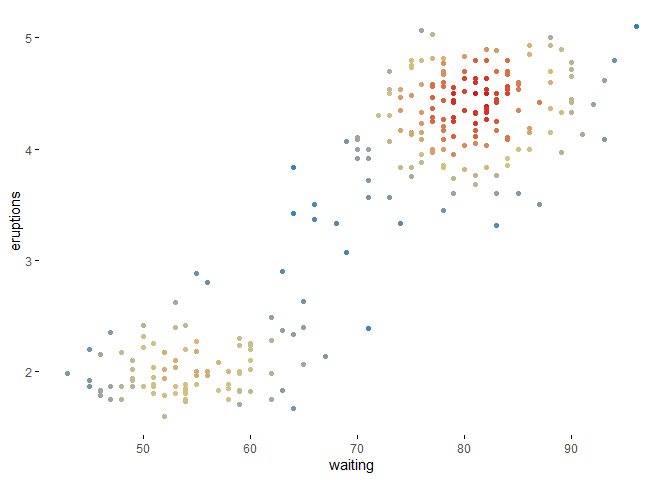This package introduces:

• wideplot() graphics for exploring the structure of a dataset through a grid of variables and graphic types.
• longplot() graphics, which present the entire catalog of available graphics for representing one particular variable or a limited number of variables using a grid of graphic types and variations on these types.
• plotup() function, which complements the previous two functions in that it presents a particular graphic for a specific variable or a limited number of variables of a dataset.

Future work will include the ability to draw longplot() and plotup() graphics from a selection of a wider number and combinations of types of variables within a dataset.

## Installation

You can install the released version of brinton from CRAN with:

install.packages("brinton")

And then load the functions included in the package:

library(brinton)
#> M a G i C i N G R a P H S

## Example

When a new dataset comes up, R users use to call the str() function that describes textually the main characteristics of this dataset. The new wideplot() function does the same but graphically. As an example, just run:

wideplot(infert)

This function includes the argument dataclass that sets the types of variables to be included in the grid as well as the order in which they are listed. As an example, the following line will plot only the numeric variables included in the iris dataset:

wideplot(infert, dataclass = c("numeric"))

Although, the wideplot() function shows only a small set of the graphics suitable for each type of data. If the user wants to see all the available graphics for some specific variable included in the dataset, then the longplot() is useful. As an example, just run:

longplot(infert, "pooled.stratum")

Any of the graphics presented in the longplot() output can also be presented. If one wants to compare the output of some other specific graphics one can, for instance, run:

wideplot(infert,
dataclass = c("numeric"),
numeric = c("point graph", "binned point graph", "binned heatmap"),
label = TRUE)

Sometimes, specially with character variables, there is not enought space for the graphic area, and the user may want to increase the width of the columns in the matrix. The user can then modify the defalut value of the ncol argument:

wideplot(infert,
dataclass = c("numeric"),
numeric = c("point graph", "binned point graph", "binned heatmap"),
label = TRUE,
ncol = 3)

If the user is interested in one particular graphic then the function plotup() is useful.

plotup(infert, "pooled.stratum", "color binned stripe graph")

Or, as example of a graphic that requires more than one input variable:

plotup(faithful, c("waiting", "eruptions"), "color scatter plot")

The default output is still a html file but the plotup() function allows to plot the graphic in the plots pane or the console:

plotup(infert, "pooled.stratum", "color binned stripe graph", output = "plots pane")plotup(faithful, c("waiting", "eruptions"), "color scatter plot", output = "plots pane")
#> Warning: Use of faithful$waiting is discouraged. Use waiting instead. #> Warning: Use of faithful$eruptions is discouraged. Use eruptions instead.plotup(infert, "pooled.stratum", "color binned stripe graph", output = "console")
#> theme_set(theme_minimal())
#>
#> binwidth <- (max(infert['pooled.stratum'], na.rm=TRUE)-min(infert['pooled.stratum'], na.rm=TRUE))/20
#> ggplot(infert, aes(x=pooled.stratum)) +
#>   geom_bin2d(aes(y=1), binwidth = c(binwidth, 1)) +
#>   scale_fill_gradientn(colours = colorRampPalette(rev(RColorBrewer::brewer.pal(4, 'Spectral')))(3)) +
#>   theme(panel.grid = element_line(colour = NA),
#>     axis.text.y =element_text(color = NA),
#>     axis.title.y =element_text(color = NA),
#>     axis.ticks.x =element_line(color = 'black'),
#>     legend.position='none')